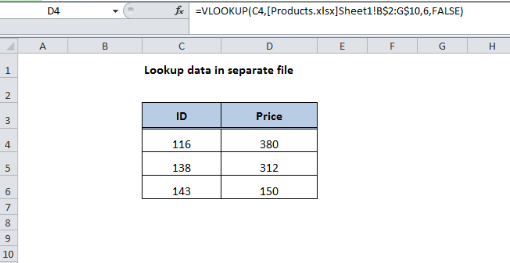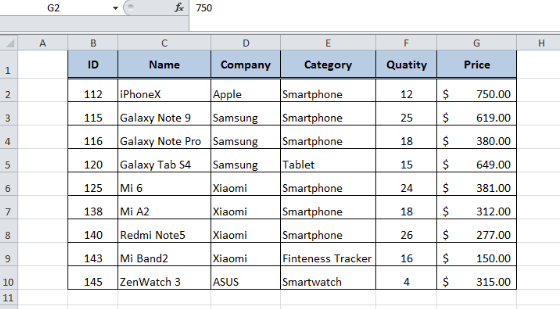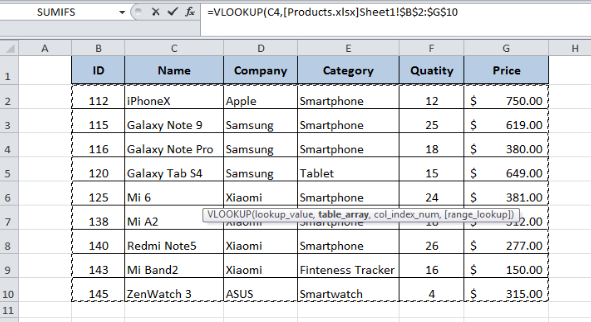Get instant live expert help with Excel or Google Sheets“My Excelchat expert helped me in less than 20 minutes, saving me what would have been 5 hours of work!”

#### Post your problem and you’ll get expert help in seconds.

Your message must be at least 40 characters
Our professional experts are available now. Your privacy is guaranteed.

# How to Use VLOOKUP from Another Workbook

Based on some unique data values we can match and retrieve the relevant information from a large dataset using various functions, such as VLOOKUP. The required information can be stored in the same worksheet, a different worksheet or even a worksheet of a completely different workbook in Excel. Here, we are going to learn how to use VLOOKUP from another workbook in Excel.Figure 1. Final result

## Formula Syntax

The generic syntax of VLOOKUP is:

=VLOOKUP(lookup_value, table_array, col_index_num, [range_lookup])

And the generic syntax of VLOOKUP from another workbook is:

=VLOOKUP(lookup_value, [workbook]Sheet! range, col_index_num, [range_lookup])

The only difference between two syntaxes is the external reference in “table_array” argument of VLOOKUP function. The syntax for external references is:

'[workbook]Sheet'! range

Where,

• Workbook: It is the name of the external workbook.
• Sheet: It is the name of the sheet in the external workbook which contains data, such as Sheet1.
• Range: It is the range of cells of the table array

Suppose we have an external workbook “Products.xlsx” in which Sheet1 contains a list of the products and their related information.Figure 2. Product list

Now, we want to retrieve information of certain products from this list using VLOOKUP formula. The best way is to enter VLOOKUP formula normally and while entering table_array argument reference the external workbook and select the range of cells of the table. In this example, we want to retrieve the price of certain products out of this products list in a separate file or workbook, say Book2 here. We will use the following formula to VLOOKUP from another workbook:

=VLOOKUP(C4,[Products.xlsx]Sheet1!B$2:G$10,6,FALSE)Figure 3. Browsing External WorkbookFigure 4. VLOOKUP formula showing results

## Explanation

The formula for VLOOKUP from another workbook is very similar to the generic VLOOKUP formula. The common identifier in both workbooks is the product ID. Therefore, we have used that ID as the lookup value. We have entered the formula in cell D4 and this formula reads as:

• Lookup for the value stored in cell C4 of the current worksheet, and use that value as a search criterion.
• Go to the external file or workbook named “Products.xlsx”,
• Then, go to the sheet named  “Sheet1” and search within the data range B2: G10.
• Then return the value stored in the 6th column of the given range (B2: G10) that is column G.

## Notes

While specifying the name of the external workbook (Products.xlsx in this case), make sure the file is present in the same directory as the workbook we are currently working on. If the external workbook is located elsewhere, we need to provide the complete path in the following format:

=VLOOKUP(C4,'\path\to\file\[Products.xlsx]Sheet1'!$B$2:$G$10,6,FALSE)

Note that it uses single quotes and includes file path, workbook name, and sheet name within the quotes. The name of the worksheet is included within these same quotes. Here’s an example:

=VLOOKUP(C4,'C:\Users\Me\Documents\records\[Products.xlsx]Sheet1'!$B$2:$G$10,6,FALSE)

## Instant Connection to an Expert through our Excelchat Service:

Most of the time, the problem you will need to solve will be more complex than a simple application of a formula or function. If you want to save hours of research and frustration, try our live Excelchat service! Our Excel Experts are available 24/7 to answer any Excel question you may have. We guarantee a connection within 30 seconds and a customized solution within 20 minutes.

### Did this post not answer your question? Get a solution from connecting with the expert.Another blog reader asked this question today on Excelchat:
Solution examplesI have a pivot table in which per order (on the rows) stands how much products they ordered per size (on the columns). I want to determine which combinations of quantities of sizes people order. And I want to count these combinations.
Solved by E. W. in 60 minsI have 500 numbers in column A with aproximatly 15digits. In column B I have 5 numbers with 6 digits Can a find a formula wich can transfer all numbers from A column wich start with some 6 digitsa from B column
Solved by V. L. in 32 minsI have a sheet with 3 columns. First column is a code for records in column B (has 563 records). What I need is a formula to show me if what is in column C (has 4400 records) is in column B and if it is to take the code for that record. Example: Column A(code corresponding to column B): 12, 14, 15, 19 Column B(names): asd, adf, ade, aqw Column C(names): akd, adf, ade, anb, wgs I need something that would say, if record in column C (for example adf) is matching record in column B (adf) return the code from column A (the code corresponding to that record in column B),if not return blank.
Solved by C. J. in 8 minsIf the data in A matches the data in C, then I need the data in E to be placed in B
Solved by X. W. in 40 minsI have a workbook with 2 sheets. First sheet has column A which is a device name, then columns B - infinity that have numeric "tags" for that device. Each device can have one or 20 "tags", tags are all numerical. Worksheet 2 has column A which is numeric value and B which is a human readable value of what the tag is. Question is, how do I replace all the tag numbers in worksheet 1 with the actual human readable values is sheet 2?
Solved by T. H. in 16 mins## Subscribe to Excelchat.coAnother blog reader asked this question today on Excelchat: